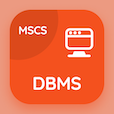# Business Statistics MCQ PDF Online

Business Statistics MCQ questions, BBA Statistics quiz questions and answers PDF to prepare online degree courses for interviews, job tests and competitive exams. "Business Statistics MCQ" PDF Book: Interquartile Range of Deviation, Mean Absolute Deviation, Squared Deviation, Relative Measure of Skewness, Classification: Measures of Dispersion, and many more topics for online learning. Practice BBA Statistics MCQ questions bank, Business Statistics quizzes from BBA Statistics textbook topics as:

## Chapters from Business Statistics Course

Practice a complete book with chapters Business Statistics Quiz Questions Bank from BBA Statistics textbooks and study notes. Following chapters are related to Business Statistics textbooks with quiz questions and answers for self-assessment as:

Confidence Intervals and Estimation Quiz
Data Classification, Tabulation and Presentation Quiz
Introduction to Probability Quiz
Introduction to Statistics Quiz
Measures of Central Tendency Quiz
Measures of Dispersion Quiz
Probability Distributions Quiz